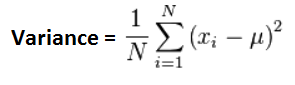﻿ Variance Calculator ﻿# Variance CalculatorEnter all numbers separated by a comma to find the variance:

This variance calculator calculates the variance of a set of numbers.

The variance is a measure of how far a set of numbers is spread out from the mean of the data set. If numbers are very spread out, it will have a high variance. If the numbers within a data set are very close together, it will have a low variance.

A variance of 0 indicates that all the values within the data set are identical. Any nonzero variance will be a positive number.

The variance is a statistical measure to show how much numbers are spread out from the average.

The square root of variance yields the standard deviation. To find the standard deviation, check out the standard deviation calculator.

To use this calculator, a user just enters in the set of numbers which he wants to compute the variance for, with each number separated by a comma. A comma must be used to separate the values, or else the calculator will not work. Any other delimiter, including a space between numbers, will cause the calculator not to work. Only commas to separate values are recognized. After the set of numbers are entered in, the user clicks the 'Calculate' button, and the resultant variance value will be calculated and displayed.

The units which the variance calculator solves for is the same as the units which are entered into the input field. For example, if the values entered into the input field are in unit inches, the resultant variance value will be in unit inches. The unit does not change from input to resultant value.

Calculating the variance is important for many various statistical purposes and provides another way to quantify our results. For electronics, we may have a average current, voltage, etc value. With variance calculated, we can determine how much variance a given current, voltage, etc is from its norm. This could be useful for various possible statistical purposes.

Related Resources

﻿